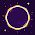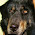### leetcode Question 111: Trapping Rain Water

Trapping Rain Water

Given n non-negative integers representing an elevation map where the width of each bar is 1, compute how much water it is able to trap after raining.
For example,
Given [0,1,0,2,1,0,1,3,2,1,2,1], return 6.The above elevation map is represented by array [0,1,0,2,1,0,1,3,2,1,2,1]. In this case, 6 units of rain water (blue section) are being trapped.

Analysis:

For the small test, we can look at the bar graph from level to level. For each level, scan from the 1st to the last, count 0s between two 1's. Add all the valid 0s for all the levels. However, if the highest and lowest bar is too much different, say 0, 100000, the loop while 100000*n, which is not efficient.

An O(n) solution is to consider each bar at a time, we can see that, for each bar, the water itself can trap depends on the max height on its left and right, e.g.  if current bar is of height 2, the max height on its left is 4, max height on its right is 3,   then water can be trapped in this bar is min(4,3)-2 = 1.

Thus, we can scan the whole map twice to get the max height on right and left, respectively. Finally scan the map again to get the water trapped of all.

Code:
class Solution {
public:
int trap(int A[], int n) {
// Start typing your C/C++ solution below
// DO NOT write int main() function
if (n<2){return 0;}

int *l = new int[n];
int *r = new int[n];

int water =0;

l=0;
for (int i=1;i<n;i++){
l[i]= max(l[i-1], A[i-1]);
}

r[n-1] = 0;
for (int i=n-2;i>=0;i--){
r[i]=max(r[i+1],A[i+1]);
}

for (int i=0;i<n;i++){
if (min(l[i],r[i])-A[i] >0 ){
water += min(l[i],r[i])-A[i];
}
}

return water;

}
};


1.2.what if how to calculate volume of water accumulated between blocks of
construction assume that block is rectangular of having length, breadth
1.